# Addition of Three 3-digit numbers with Regrouping | Maths Grade 2

### Addition of Three 3-digit numbers with Regrouping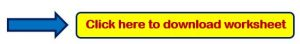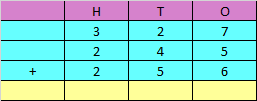Explanation:

Step 1: Adding the digits at one’s place,

7 + 5 + 6 = 18

Since the number is more than 9, we need to carry over 1 to the tens place.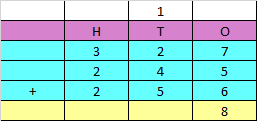Step 2: Adding the digits at tens place and the carry of 1,

2 + 4 + 5 + 1 = 12

Since the number is more than 9, we need to carry over 1 to the hundreds place.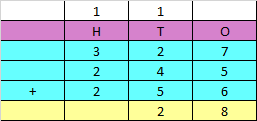Step 3: Adding the digits at hundreds place and carry 1,

3 + 2 + 2 + 1 = 8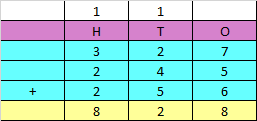Hence, the sum of the given numbers is 828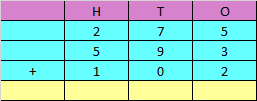Step 1: Adding the digits at one’s place,

5 + 3 + 2 = 10

Since the number is more than 9, we need to carry over 1 to the tens place.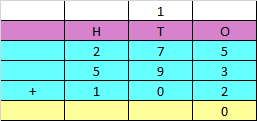Step 2: Adding the digits at tens place and the carry of 1,

7 + 9 + 0 + 1 = 17

Since the number is more than 9, we need to carry over 1 to the hundreds place.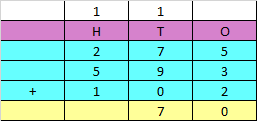Step 3: Adding the digits at hundreds place and carry 1,

2 + 5 + 1 + 1 = 9Hence, the sum of the given numbers is 970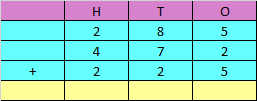Explanation:

Step 1: Adding the digits at one’s place,

5 + 2 + 5 = 12

Since the number is more than 9, we need to carry over 1 to the tens place.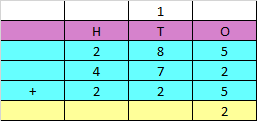Step 2: Adding the digits at tens place and the carry of 1,

8 + 7 + 2 + 1 = 18

Since the number is more than 9, we need to carry over 1 to the hundreds place.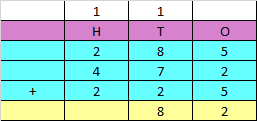Step 3: Adding the digits at hundreds place and carry 1,

2 + 4 + 2 + 1 = 9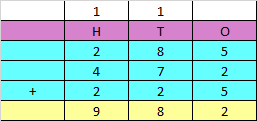Hence, the sum of the given numbers is 982